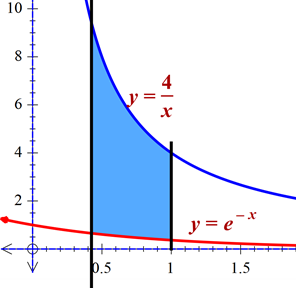# Sketch the region bounded by the graphs of the functions. \displaystyle f(x) = \frac{4}{x}, g(x)...

## Question:

Sketch the region bounded by the graphs of the functions.

{eq}\displaystyle f(x) = \frac{4}{x}, g(x) = -e^x, x = \frac{1}{2}, x = 1 {/eq}.

Find the area of the region.

## Answer and Explanation:To find the area bounded by the curves we will use the formula:

{eq}=\int (f(x)-g(x))dx {/eq}

Here f(x) is the curve {eq}=\frac{4}{x}\\ g(x)=-e^{x} {/eq}

Now the area becomes:

{eq}=\int_{\frac{1}{2}}^{1}(\frac{4}{x}+e^{x})dx\\ =\left [ 4\ln x+e^{x} \right ] {/eq}

Now plug-in the upper and the lower bounds:

{eq}=e+4\ln 2-e^{\frac{1}{2}} {/eq}

#### Learn more about this topic:

What is Area in Math? - Definition & Formula

from ELM: CSU Math Study Guide

Chapter 12 / Lesson 8
190K